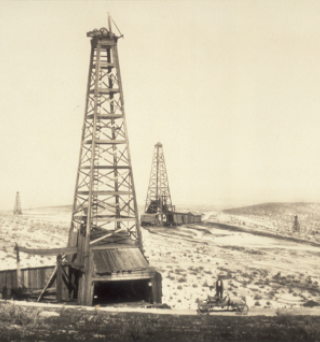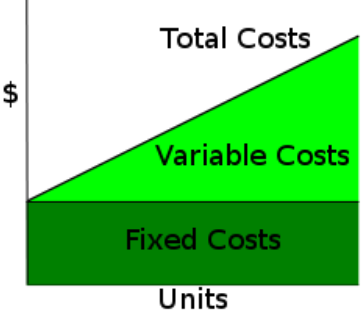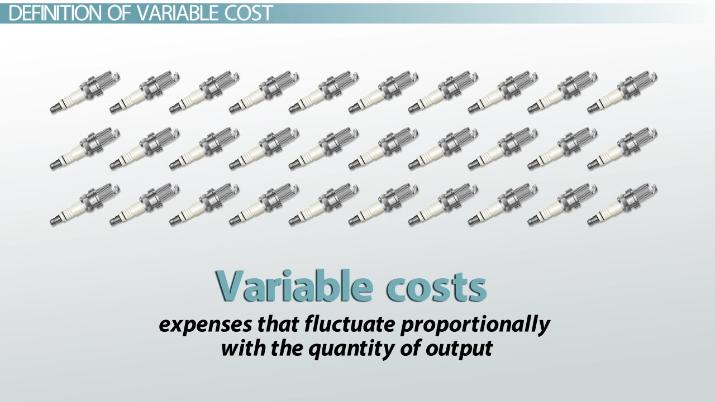# Variable Cost Formula in Business Accounting

Jack Woerner, James Carnrite, Steven Scalia
• Author
Jack Woerner

BA in Political Science with Emphasis on Social Studies Education at Brevard College, 6 years experience (2 years online) teaching Economics, Personal Finance, APUS Government and more. Certified Gifted/Talented Teacher.

• Instructor
James Carnrite

James has an MBA in IT Management.

• Expert Contributor
Steven Scalia

Steven completed a Graduate Degree is Chartered Accountancy at Concordia University. He has performed as Teacher's Assistant and Assistant Lecturer in University.

Understand variable cost in business. Learn the definition of variable cost, the variable cost formula, and how to use the formula to calculate the variable cost. Updated: 02/15/2022

Show

## Variable Cost

The variable cost definition in business accounting means any expense that changes dependent on the amount of products being produced. Variable costs can increase in one month and then be lower the next month depending on the business's overall production. A general business rule for variable costs is if a production is high, variable costs are high and vice versa. Variable cost involves indirect and direct cost. Direct costs are costs that are directly related to the production of a product. Indirect costs are expenses that are not directly related to the production process but are related to the servicing of the product during or after its produced. Businesses use a variable cost formula to calculate for variable cost.

### Examples of Variable Cost

There are many variable cost examples, and variable costs are unique to each business. Common variable cost examples seen in businesses include:

• Labor
• Utilities (energy and water)
• Supplies
• Shipping and handling costs
• Marketing
• Sales related costs
• Transaction fees like bank fees and credit card fees
• Raw materials### Fixed Cost vs Variable Cost

Fixed costs differ from variable costs. The fixed cost definition in accounting describes expenses that stay constant no matter how much is being produced. Fixed costs will remain the same when business activity is high or low. Fixed costs are not usually direct costs that are involved in the production process. Variable costs are usually consumable items that changes when the volume of production changes.

Common fixed cost examples include:

• Rent on property
• Insurance
• Storage fees
• Loan payments
• Equipment rentals
• Asset depreciation

### Total Cost

Total cost is the final total costs to produce a product during a set time period. To find total costs, a business must find the total variable costs and fixed costs. The total cost formula is Variable costs + Fixed Costs = Total cost.

For example:

An apple orchard business spent \$10,000 on fixed costs for the month of September. In the same month, their variable costs totaled to be 27,532. The apple orchard accountant wants to calculate for the total costs before the end of the season.

10,000 + 27,532 = \$37,532 in total costs for the month of September.### Variable Cost Formula

To find variable cost, a business must use the variable cost formula. To find variable cost a business must find the cost per unit and the output quantity in the time period in which they are calculating for.

The variable cost formula is: Total variable cost = total quantity of output X variable cost per unit of output

For example: A start-up company makes 100 products in one month and it each product costs \$5.00 per unit. The business wants to sell these products for \$20 a piece. To calculate for variable cost this month, the formula would like: 100 x 5 = \$500 total variable cost.

### Fixed Cost Formula

The fixed cost equation is: Fixed costs = Total cost of production - (Variable cost per unit x Number of units produced). In order to calculate for fixed costs, the a business must:

1. Add up all the costs in the time period they are targeting.
2. Organize fixed costs and variable costs.
3. Take the variable cost of each unit multiplied by the number of units produced.
4. Find fixed costs bytaking the total costs of production minus the variable cost found in step three.

### Total Cost Formula

The sum of the variable cost and the fixed cost formulas yields the total cost formula.

The total cost formula is: Total cost = Total Variable Cost + Total Fixed Cost

Total cost calculation example: A business experiences \$50,000 total variable costs and \$25,000 fixed costs for one month of business activity. To find total cost: 50,000 + 25,000 = \$75,000 for total costs in that month.An error occurred trying to load this video.

Try refreshing the page, or contact customer support.

Coming up next: Accounting Disciplines: Descriptions and Definitions

### You're on a roll. Keep up the good work!

Replay
Your next lesson will play in 10 seconds
• 0:01 Definition of Variable Cost
• 0:42 Formula of Variable Cost
• 4:07 Lesson Summary
Save Save

Want to watch this again later?

Timeline
Autoplay
Autoplay
Speed Speed

## Average Variable Cost

The average variable cost definition is the variable cost per unit.

The formula to find the variable cost per unit is: Average Cost Per Unit = Variable Cost / Quantity produced

For example: A business that has \$400 in variable cost for one month and is producing 20 products in that month would experience \$20 variable cost per unit. 400/20 = 20.

## Average Fixed Cost

The average fixed cost definition is the fixed cost per unit produced.

To unlock this lesson you must be a Study.com Member.

• Activities
• FAQs

## Variable Cost - A Practical Exercise

The following exercise is designed to help students apply their knowledge of variable cost and its formula in a real-life scenario.

### Scenario

You are a management consultant specializing in cost management. Your newest client, Beauty Ice Cream ("Beauty"), is a local ice cream shop that sells homemade, artisanal ice cream in a small location just off the college campus in Cambridge. Beauty is looking for help because it wants to better understand its cost structure. You have the following voice notes from your interview with Milky Dairy, the owner of the shop.

"You would think an ice cream shop is a simple business, but we cannot turn a profit. It's driving me insane. I need some help understanding my cost structure so that I can make better decisions."

"We don't have that many costs: We buy the ice cream in bulk from a local ice cream maker, it comes out to roughly \$0.40 per scoop. We then buy our cones at \$1 for a pack of 10 units. We add our special frosty sprinkles, which cost \$0.10 per scoop on average. Besides that, our rent is \$900 per month, or \$30 per day. We pay our only employee \$10 per hour for 8 hours, or \$80 per day. We sign an annual contract with her and I would never fire her. That's all we have."

### Required

1. What is the total variable cost per unit (assuming one scoop per cone sold)?

2. Determine the variable cost function of Beauty.

3. If the company were to sell 500 units, how much would its total variable cost be?

### Solution

1. See below.

Item\$
Ice cream0.40
Sprinkles0.10
Cones =\$1/100.10
Total variable cost\$0.60

The other costs are fixed in nature.

The answer is thus 60 cents per unit.

2. Total variable cost = Ice cream cones sold * \$0.60

3. Total variable cost = Ice cream cones sold * \$0.60

= 500 * 0.60

=\$300

#### What is a variable cost example?

A variable cost example is anything directly related and consumed during production. Raw materials, labor, and supplies are common variable cost examples.

#### How do you calculate fixed and variable costs?

Fixed costs = Total cost of production - (Variable cost per unit x Number of units produced). Variable cost = total quantity of output X variable cost per unit of output

#### What is the formula for variable cost?

The formula for variable costs is: total quantity of output X variable cost per unit of output = variable cost. A business would need to find this data for a set time period.

#### What are some examples of fixed and variable costs?

Fixed cost examples are expenses like rent, storage, and insurance fees. Variable costs examples are direct labor, materials, supplies, and energy used in the production process.

#### What is the formula of variable cost per unit?

The variable cost per unit formula is the same as the average variable cost formula. It is Average Cost Per Unit = Variable Cost / Quantity produced.

### Register to view this lesson

Are you a student or a teacher?